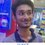# Can this be the solution for composites?

Propositions:

It is well known by the standard Riemann Zeta function that

$\zeta (s) = \displaystyle \sum_{n=1}^{\infty} \dfrac{1}{n^s}$

For $\mathfrak{R} (s) > 1$.

Also, the Prime Zeta function gives us

$P(s) = \displaystyle \sum_{p} \dfrac{1}{p^s}$

For $p \in \{ \text{primes} \}$ and $\mathfrak{R} (s) > 1$.

Conclusion:

This means that the sum of $s^{\text{th}}$ powers of the reciprocals of all composite numbers can be given by the formula

\begin{aligned} P'(s) &= \displaystyle \sum_{p'} \dfrac{1}{{p'}^s} \\ &= \sum_{n=1}^{\infty} \dfrac{1}{n^s} - \sum_{p} \dfrac{1}{p^s} - 1 \\ &= \zeta (s) - P(s) - 1 \end{aligned}

For $p' \in \{ \text{composite numbers} \}$ and $\mathfrak{R} (s) > 1$.

Knowing that infinite sums are risky to handle. I would like your opinions on whether the conclusion drawn from the two given true propositions made above are correct or not.Note by Tapas Mazumdar
4 years, 4 months ago

This discussion board is a place to discuss our Daily Challenges and the math and science related to those challenges. Explanations are more than just a solution — they should explain the steps and thinking strategies that you used to obtain the solution. Comments should further the discussion of math and science.

When posting on Brilliant:

• Use the emojis to react to an explanation, whether you're congratulating a job well done , or just really confused .
• Ask specific questions about the challenge or the steps in somebody's explanation. Well-posed questions can add a lot to the discussion, but posting "I don't understand!" doesn't help anyone.
• Try to contribute something new to the discussion, whether it is an extension, generalization or other idea related to the challenge.

MarkdownAppears as
*italics* or _italics_ italics
**bold** or __bold__ bold
- bulleted- list
• bulleted
• list
1. numbered2. list
1. numbered
2. list
Note: you must add a full line of space before and after lists for them to show up correctly
paragraph 1paragraph 2

paragraph 1

paragraph 2

[example link](https://brilliant.org)example link
> This is a quote
This is a quote
    # I indented these lines
# 4 spaces, and now they show
# up as a code block.

print "hello world"
# I indented these lines
# 4 spaces, and now they show
# up as a code block.

print "hello world"
MathAppears as
Remember to wrap math in $$ ... $$ or $ ... $ to ensure proper formatting.
2 \times 3 $2 \times 3$
2^{34} $2^{34}$
a_{i-1} $a_{i-1}$
\frac{2}{3} $\frac{2}{3}$
\sqrt{2} $\sqrt{2}$
\sum_{i=1}^3 $\sum_{i=1}^3$
\sin \theta $\sin \theta$
\boxed{123} $\boxed{123}$

Sort by:

if $u_n,v_n$ are convergent sequences then so is their difference. Provided $\zeta(s),P(s)$ converges which is obvious ,your conclusions are true enough

- 4 years, 3 months ago